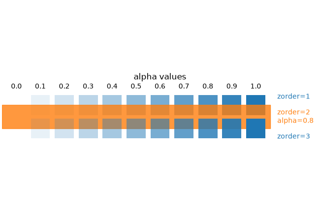# matplotlib.colors.to_rgba_array#

matplotlib.colors.to_rgba_array(c, alpha=None)[source]#

Convert c to a (n, 4) array of RGBA colors.

Parameters:
cMatplotlib color or array of colors

If c is a masked array, an `ndarray` is returned with a (0, 0, 0, 0) row for each masked value or row in c.

alphafloat or sequence of floats, optional

If alpha is given, force the alpha value of the returned RGBA tuple to alpha.

If None, the alpha value from c is used. If c does not have an alpha channel, then alpha defaults to 1.

alpha is ignored for the color value `"none"` (case-insensitive), which always maps to `(0, 0, 0, 0)`.

If alpha is a sequence and c is a single color, c will be repeated to match the length of alpha.

Returns:
array

(n, 4) array of RGBA colors, where each channel (red, green, blue, alpha) can assume values between 0 and 1.

## Examples using `matplotlib.colors.to_rgba_array`#Specifying colors

Specifying colors